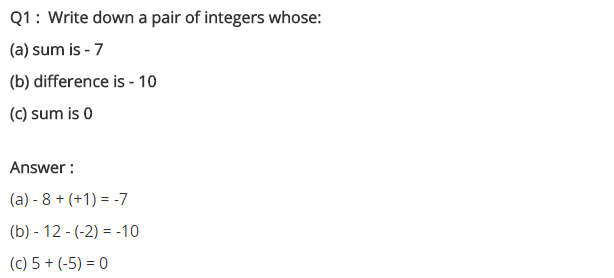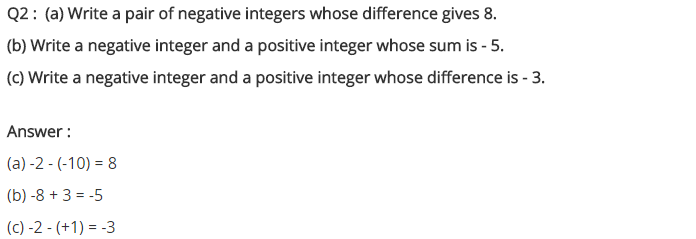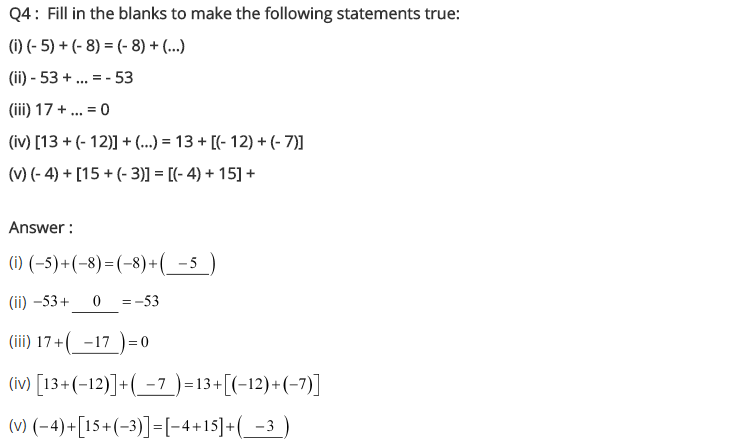# NCERT Solutions for Class 7 Maths Chapter 1 Integers Ex 1.2

NCERT Solutions for Class 7 Maths Chapter 1 Integers Exercise 1.2
Ex 1.2 Class 7 Maths Question 1.
Write down a pair of integers whose:
(a) sum is -7
(b) difference is -10
(c) sum is 0.
Solution:
(a) Let us take a pair of integers -3 and -4.
∴ (-3) + (-4) = -3 – 4 = -7
(b) Let us take a pair of integers -12 and -2
∴ (-12) – (-2) = -12 + 2 = -10
(c) Let us take a pair of integers -3 and 3
∴ (-3) + (3) = -3 + 3 = 0

Ex 1.2 Class 7 Maths Question 2.
(a) Write a pair of negative integers whose difference gives 8.
(b) Write a negative integer and positive integer whose sum is -5.
(c) Write a negative integer and a positive integer whose difference is -3.
Solution:
(a) Let us have -2 and -10
∴ Difference = (-2) – (-10) = -2 + 10 = 8
(b) Let us have -7 and 2
∴ (-7) + (2) = -7 + 2 = -5
(c) Let us have -2 and 1
∴ (-2) – (1) = – 2 – 1 = -3

Ex 1.2 Class 7 Maths Question 3.
In a quiz, team A scored -40, 10, 0 and team B scored 10, 0, -40 in three successive rounds. Which team scored more? Can you say that we can add integers in any order?
table
Solution:
Total score of team
A = (-40) + (10) + (0) – -40 + 10 + 0 = -30
Total score of team
B = 10 + 0 + (-40) = 10 + 0 – 40 – -30
∴ The scores of both the teams are same i.e. -30.
Yes, we can add the integers in any order.

Ex 1.2 Class 7 Maths Question 4.
Fill in the blanks to make the following statements true:
(i) (-5) + (-8) = (-8) + (…)
(ii) -53 + … = -53
(iii) 17 + … = 0
(iv) [13 + (-12)] + (…) = 13 + [(-12) + (-7)]
(v) (-4) + [15 + (-3)] = [-4 + 15] + …
Solution:
(i) -5 + (-8) – (-8) + (-5) [Commutative law of additions]
(ii) -53 + 0 = -53 [Additive Identity]
[Adding 0 to any integer, it gives the same value]
(iii) 17 + (-17) = 0 [Additive inverse]
(iv) [13 + (-12)] + (-7) – 13 + [(-12) + (-7)] [Associative law of addition]
(v) (-4) + [15 + (-3)] – [-4 + 15] + (-3) [Associative law of addition]+